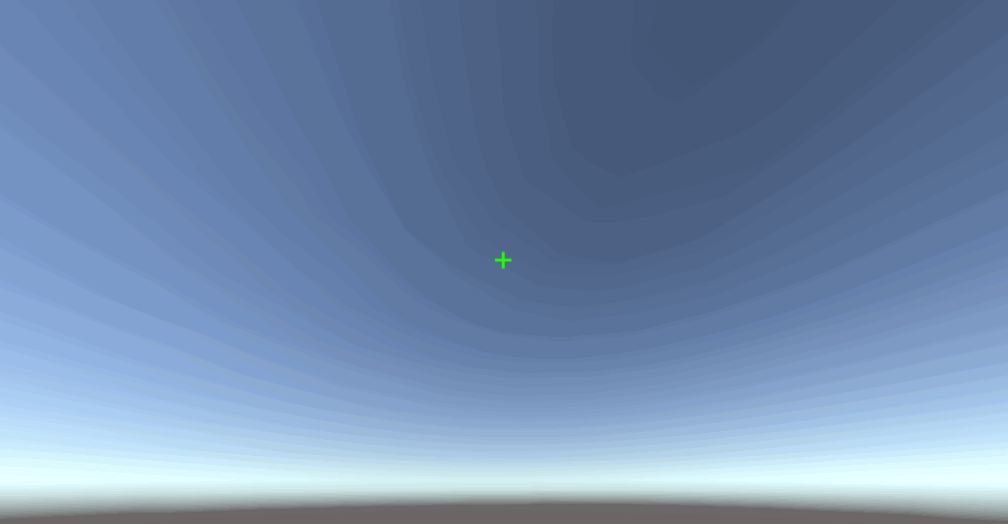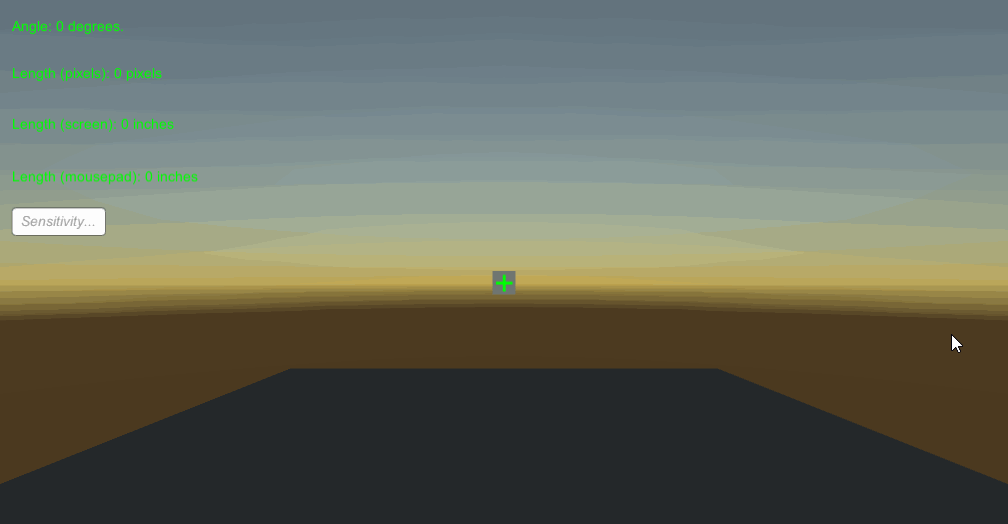# Calculating Distance Per 360 Sensitivity in Unity3D

Posted on Friday 23-February-2018

Physical mouse distance to camera view movement can be an important attribute in some projects. For particular games (primarly aim training games like Kovaak’s), it is important to be able to measure the physical mouse distance in relation to the camera. Most commonly, this calculation is refered to as the inches/cm per 360 sensitivity, and can be a useful metric in unfying look sensitivity between games. There exist entire sites dedicated to making this conversion.

The scripts in this post will be Unity-specific, however the process should be able to be easily applied to any engine.

## Rotation Degrees

The first step is obviously a script that lets the mouse affect the camera. A basic implementation might look like the following:

``````using UnityEngine;
using System.Collections;
using System.Collections.Generic;

public class MouseLook : MonoBehaviour {
public float sensitivityX = 1f;
public float sensitivityY = 1f;
public float minimumX = -360f;
public float maximumX = 360f;
public float minimumY = -60f;
public float maximumY = 60f;
private float rotationX = 0f;
private float rotationY = 0f;
private Quaternion originalRotation;

private void Update () {
//Gets rotational input from the mouse
rotationY += Input.GetAxisRaw("Mouse Y") * sensitivityY;
rotationX += Input.GetAxisRaw("Mouse X") * sensitivityX;

//Clamp the rotation average to be within a specific value range
rotationY = ClampAngle(rotationY, minimumY, maximumY);
rotationX = ClampAngle(rotationX, minimumX, maximumX);

//Get the rotation you will be at next as a Quaternion
Quaternion yQuaternion = Quaternion.AngleAxis(rotationY, Vector3.left);
Quaternion xQuaternion = Quaternion.AngleAxis(rotationX, Vector3.up);

//Rotate
transform.localRotation = originalRotation * xQuaternion * yQuaternion;
}

private void Start () {
originalRotation = transform.localRotation;
}

public static float ClampAngle (float angle, float min, float max) {
angle = angle % 360;
if ((angle >= -360f) && (angle <= 360f)) {
if (angle < -360f) {
angle += 360f;
}
if (angle > 360f) {
angle -= 360f;
}
}
return Mathf.Clamp (angle, min, max);
}
}
``````

One irregularity here is that we’re using `Input.GetAxisRaw()` rather than `Input.GetAxis()`. To be honest, I haven’t observed any differences between the two in regards to mouse input. Allegedly, the former has no smoothing filtering applied, which is typically paramount if aim is important in your game. Smoothing can always be implemented yourself, and in a much more controllable way than using Unity’s inbuilt smoothing.

There are a couple of niceties in this script that will make our life easier. In particular, notice `rotationX`. This variable is our rotation angle from the center in degrees; `rotationX` will be 180 when we’re looking directly behind our camera’s original position and 0 when we’re looking straight ahead. Using this variable, we can tell how far the camera is turned – a step closer to calculating physical distance per 360 degrees.## Physical Cursor Distance

While physical cursor distance isn’t what this post is about, it’s valuable in aiding the conversion. It’s also beneficial in understanding some of the key concepts here.

### Screen DPI

Screen DPI (dots per inch, but also pixels per inch) defines how many pixels are within each inch of space on the display. A list of common device DPI values and a calculator can be found here. Screen DPI helps us correlate traveling 1 pixel to a physical distance on the display. For a display of 96 DPI, when the cursor moves 1 pixel, it moves 1/96th of an inch – 0.010416”.

### Application

To apply this to our project, we can use Unity’s `Screen.dpi` property together with a mouse delta value to figure out the physical distance our cursor has traveled on the real life screen;

``````float mouseXDelta = 0f;
float cursorDistancePixels = 0f;
float cursorDistanceInches = 0f;

// Get the mouse delta -- 0.05 = 1 pixel worth of movement, so multiply by 20.
mouseXDelta = Input.GetAxisRaw("Mouse X") * 20f;
cursorDistancePixels += Mathf.Abs(mouseXDelta);

// Figure out how many physical inches the mouse cursor has traveled.
cursorDistanceInches = cursorDistancePixels / Screen.dpi;
``````

Notice that we multiply Unity’s mouse delta result by 20. This is so that the value corresponds to 1 pixel = a delta of 1. The value of `GetAxis()` and `GetAxisRaw()` is multiplied by the mouse axis’ sensitivity value set by Unity’s input manager (Edit -> Project Settings -> Input). My version of Unity defaulted to 0.1, which somehow equates to a value of 0.05 being equal to 1 pixel worth of movement, so that’s the basis I’ll use for the rest of the post. To avoid multiplying by 20, you could also just set the sensitivity Unity uses to 2.

This delta value only shows change when the mouse moves at least a pixel, so higher resolution displays should be more accurate in theory. If you want true delta, you’ll have to access it through the OS – here’s a good starting point for Windows.

Note: I’ve heard that the value from `GetAxis()` / `GetAxisRaw()` can vary depending on mouse. I haven’t seen this behaviour myself, and it doesn’t make sense that it should, but it’s something to be careful of nonetheless.

## Physical Mouse Distance

### Mouse DPI

Mouse DPI defines how many movements are measured over an inch. At 400 DPI and assuming a perfect sensor / infinite polling rate, 400 instructions are reported to the OS for every inch of movement. In reality it doesn’t always work quite like this, but for our purposes we needn’t bother with more specifics.

It then follows that we should be able to simply divide the mouse delta by our dpi to achieve a physical distance.

### Application

The application here is much the same as calculating mouse cursor distance;

``````float mouseXDelta = 0f;
float cursorDistancePixels = 0f;
float mouseDistanceInches = 0f;
const float mouseDPI = 800f;

// Get the mouse delta -- 0.05 = 1 pixel worth of movement, so multiply by 20.
// Why?  I don't know.
mouseXDelta = Input.GetAxisRaw("Mouse X") * 20f;
cursorDistancePixels += Mathf.Abs(mouseXDelta);

// Figure out how many physical inches the mouse has traveled.
mouseDistanceInches = cursorDistancePixels / mouseDPI;
``````

Here, `mouseDPI` represents the DPI of the player’s mouse. Unfortunately, I know of no programmatic way get mouse DPI, and there probably is no way due to its nature – we know the monitor DPI because it is reported over the interface (HDMI / DisplayPort / etc), but mice have no such device. This is something you’ll have to query players about.

## Tying Everything Together

To accomplish our original goal of calculating mouse distance per a 360 degree rotation, we just need to quickly revise our initial mouse script.

``````public class MouseLook : MonoBehaviour {
public float mouseDPI = 800f;

public float sensitivityX = 15f;
public float sensitivityY = 15f;
public float minimumX = -360f;
public float maximumX = 360f;
public float minimumY = -60f;
public float maximumY = 60f;
private float rotationX = 0f;
private float rotationY = 0f;
private Quaternion originalRotation;

private float mouseXPos;
private float cursorTotalDelta = 0f;
private float mouseDistanceInches = 0f;
private float stop = false;

private void Update () {
//Gets rotational input from the mouse
rotationY += Input.GetAxisRaw("Mouse Y") * sensitivityY;
mouseXDelta = Input.GetAxisRaw("Mouse X");
rotationX += mouseXDelta * sensitivityX;

// If we haven't reached 360 degrees yet...
if (!stop)
cursorTotalDelta += Mathf.Abs(mouseXDelta * 20f);
mouseDistanceInches = cursorTotalDelta / mouseDPI;

//Clamp the rotation average to be within a specific value range
rotationY = ClampAngle(rotationY, minimumY, maximumY);
// If we've rotated 360 degrees, stop adding to the total distance.
if (rotationX >= 360f) {
rotationX = ClampAngle(rotationX, minimumX, maximumX);
stop = true;
}

//Get the rotation you will be at next as a Quaternion
Quaternion yQuaternion = Quaternion.AngleAxis(rotationY, Vector3.left);
Quaternion xQuaternion = Quaternion.AngleAxis(rotationX, Vector3.up);

//Rotate
transform.localRotation = originalRotation * xQuaternion * yQuaternion;
}

private void Start () {
originalRotation = transform.localRotation;
}

public static float ClampAngle (float angle, float min, float max) {
angle = angle % 360;
if ((angle >= -360f) && (angle <= 360f)) {
if (angle < -360f) {
angle += 360f;
}
if (angle > 360f) {
angle -= 360f;
}
}
return Mathf.Clamp (angle, min, max);
}
}
``````

Now `mouseDistanceInches` holds the mouse distance in inches after a 360 degree turn. As mentioned earlier, due to Unity’s frame-by-frame nature this value won’t be 100% on the nose, particularly if the mouse is moved quickly near the end of the rotation. Logically, this is due to a high mouse delta being buffered over a frame. That is, the mouse is moving faster than frames are being rendered. If you require extreme (mid-frame) accuracy, look towards a framerate independent solution: http://www.sophiehoulden.com/super-fast-input-in-unity/.

In the Unity project (linked at the bottom of the post), I’ve set up a nice debug UI that should make it easy to see what’s going on with the various values.## Solving For Distance Mathematically

Of course, once we’ve figured out the angle that a mouse delta of 1 relates to, we can solve distance for 360 degrees. If we set our look sensitivity to 1 (in the Unity inspector) so that it doesn’t interfere with our mouse delta, we can use our current system to find that – with the rotation method we’re using – 20 dots of mouse movement is equal to 1 degree of rotation.With this information we can associate 1 delta worth of movement with 0.05 degrees of rotation, when using a sensitivity of 1. Given this, the following equation should calculate distance for a 360 given any sensitivity:

(360 / (sensitivity * 0.05)) / DPI = mouse distance in inches

And to do the same calculation for sensitivity rather than distance:

mouse distance in inches * DPI / (360 / 0.05) = sensitivity

And there you have it! Physical mouse distance per 360 accurately calculated within Unity. These scripts and formulas should work uniformly, though I have by no means done any extensive testing.
Hopefully through reading this blog post you have gained the understanding to solve this problem for any engine, and fix any minor issues that may arise in the scripts posted here due to a Unity version change or otherwise.

Full scripts available at this github repository.

Discuss on GitHub